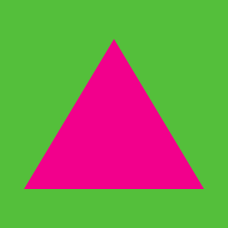Geometry

# Triangles - Angle SumIn the above triangle, the blue angle is $30^\circ$ and the green angle is $70 ^ \circ$. What is the measure of the yellow angle (in degrees)?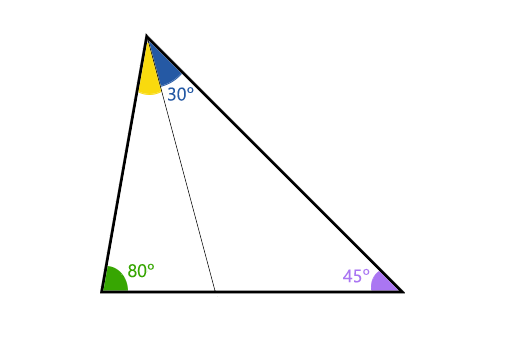In the above triangle, the blue angle is $30^\circ$, the green angle is $80 ^ \circ$, and the purple angle is $45 ^ \circ$. What is the measure of the yellow angle?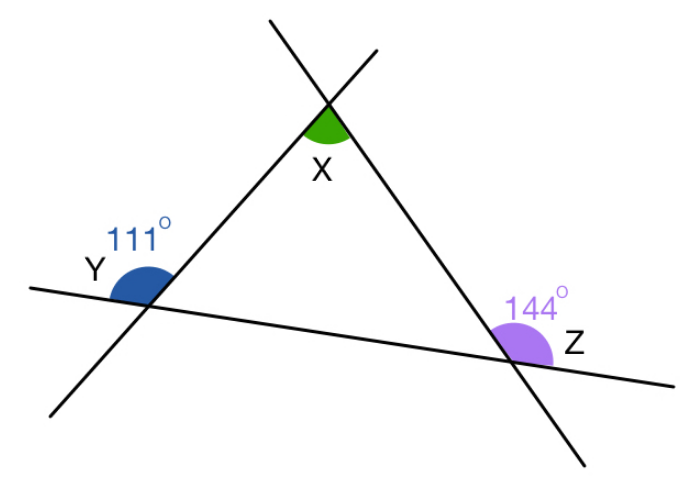In the above diagram, the purple angle at $Z$ equals $\color{#69047E}{ 144}^\circ$ and the blue angle at $Y$ equals $\color{#3D99F6} {111} ^ \circ$. What is the measure of the green angle at $X$?

Note: The diagram is not drawn to scale.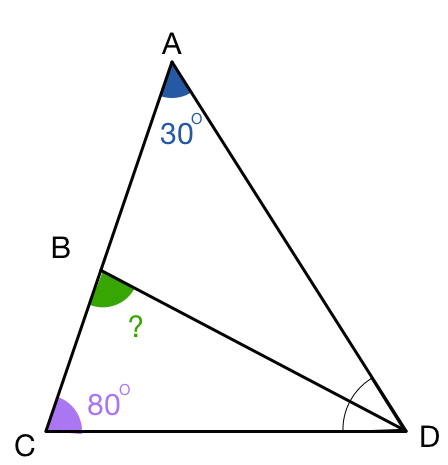In the above diagram, $\color{#3D99F6} {\angle CAD=30^\circ}, \color{#69047E}{\angle ACD=80^\circ}$ and line $\overline{BD}$ is a bisector of $\angle ADC$. What is $\color{#20A900}{\angle CBD}$ in degrees?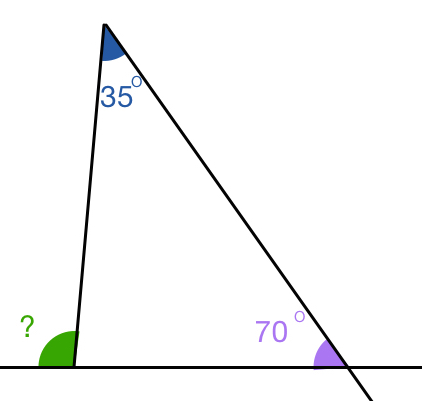In the above diagram, what is $\color{#20A900} {\text{green angle}}$ in degrees?

×